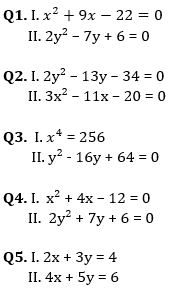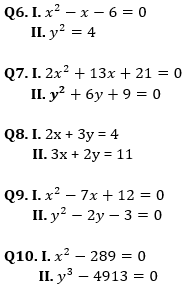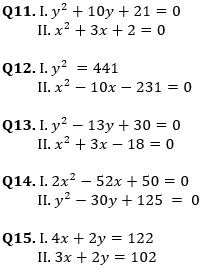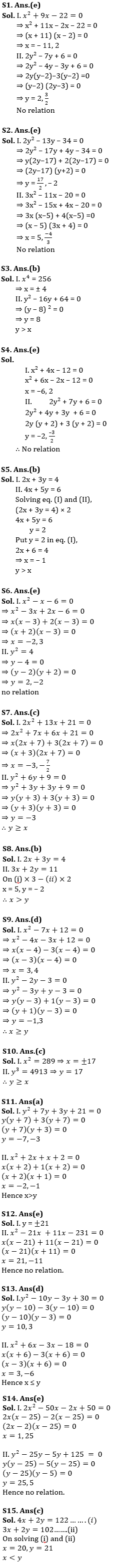Latest Banking jobs   »   Quantitative Aptitude Quiz For IRDA AM...

# Quantitative Aptitude Quiz For IRDA AM 2023-03rd May

Directions (1-5): In the following questions there are two equations given. You have to solve both the equations and give answer:
(a) if x > y
(b) if x < y
(c) if x ≥ y
(d) if x ≤ y
(e) if x = y or relation between x and y cannot be establishedDirections (6-10): Two equations I and II are given below in each question. You have to solve these equations and give answer accordingly.
(a) if x < y
(b) if x > y
(c) if x ≤ y
(d) if x ≥ y
(e) if x = y or no relation can be establishedDirections (11 – 15): In the following questions, two equations, I and II are given. You have to solve both the equations and give answer accordingly.
(a) if x > y
(b) if x ≥ y
(c) if x < y
(d) if x ≤ y
(e) if x = y or no relation.Solutions## FAQs

### How many sections are there in the IRDAI exam?

There are 4 sections in the IRDAI assistant manager online preliminary exam. They are Reasoning, English Language, General awareness, Quantitative aptitude.

#### Congratulations!Union Budget 2023-24: Free PDF